# 1077 functions are available on our servers and librariesidiv, irem (division with remainder), ishift (bit shifting), iand, ior, ixor (logical operations), igcd,(GCD by various methods such as Euclid's algorithm and the accelerated GCD algorithm), fac (factorial), inv (inverse modulo an integer), random (random number generator by the Mersenne twister algorithm).Arithmetics on various fields: the rationals,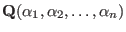(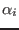is algebraic over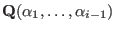),(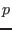is a prime of arbitrary size),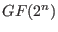.sdiv , srem (division with remainder), ptozp (removal of the integer content), diff (differentiation), gcd (GCD over the rationals), res (resultant), subst (substitution), umul (fast multiplication of dense univariate polynomials by a hybrid method with Karatsuba and FFT+Chinese remainder), urembymul_precomp (fast dense univariate polynomial division with remainder by the fast multiplication and the precomputed inverse of a divisor),fctr (factorization over the rationals), fctr_ff (univariate factorization over finite fields), af (univariate factorization over algebraic number fields), sp (splitting field computation).dp_gr_main (Groebner basis computation of a polynomial ideal over the rationals by the trace lifting), dp_gr_mod_main (Groebner basis over small finite fields), tolex (Modular change of ordering for a zero-dimensional ideal), tolex_gsl (Modular rational univariate representation for a zero-dimensional ideal), dp_f4_main (over the rationals), dp_f4_mod_main (over small finite fields).primedec (Prime decomposition of the radical), primadec (Primary decomposition of ideals by Shimoyama/Yokoyama algorithm).qe (real quantifier elimination in a linear and quadratic first-order formula), simpl (heuristic simplification of a first-order formula).

 MTP2 = ex([x11,x12,x13,x21,x22,x23,x31,x32,x33],
x11+x12+x13 @== a1 @&& x21+x22+x23 @== a2 @&& x31+x32+x33 @== a3
@&& x11+x21+x31 @== b1 @&& x12+x22+x32 @== b2 @&& x13+x23+x33 @== b3
@&& 0 @<= x11 @&& 0 @<= x12 @&& 0 @<= x13 @&& 0 @<= x21
@&& 0 @<= x22 @&& 0 @<= x23 @&& 0 @<= x31 @&& 0 @<= x32 @&& 0 @<= x33)$ TSOL= a1+a2+a3@=b1+b2+b3 @&& a1@>=0 @&& a2@>=0 @&& a3@>=0 @&& b1@>=0 @&& b2@>=0 @&& b3@>=0$
 QE_MTP2 = qe(MTP2)\$
 qe(all([a1,a2,a3,b1,b2,b3],QE_MTP2 @equiv TSOL));
@trueplot (plotting of a univariate function), ifplot (plotting zeros of a bivariate polynomial), conplot (contour plotting of a bivariate polynomial function).det (determinant), qsort (sorting of an array by the quick sort algorithm), eval (evaluation of a formula containing transcendental functions such as sin, cos, tan, exp, log) roots (finding all roots of a univariate polynomial), lll (computation of an LLL-reduced basis of a lattice).

http://www.openxm.org

Nobuki Takayama 2017-03-30